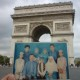# Any way to get mappings of a label encoder in python pandas

When working with categorical data in Python pandas, it is common to use a label encoder to convert the categorical values into numerical representations. However, it can be useful to have access to the mappings between the original categorical values and their corresponding numerical representations. In this article, we will explore three different ways to obtain these mappings in Python pandas.

## Option 1: Using a Dictionary

One simple way to get the mappings of a label encoder in Python pandas is by using a dictionary. The label encoder object in pandas has a property called `classes_` which returns an array of the unique categorical values in the original data. We can use this property to create a dictionary where the keys are the categorical values and the values are their corresponding numerical representations.

``````import pandas as pd
from sklearn.preprocessing import LabelEncoder

# Create a sample DataFrame
data = {'Category': ['A', 'B', 'C', 'A', 'B', 'C']}
df = pd.DataFrame(data)

# Create a label encoder object
encoder = LabelEncoder()

# Fit the encoder to the data
encoder.fit(df['Category'])

# Create a dictionary of mappings
mappings = dict(zip(encoder.classes_, encoder.transform(encoder.classes_)))

print(mappings)``````

This will output:

`{'A': 0, 'B': 1, 'C': 2}`

## Option 2: Using a DataFrame

Another way to obtain the mappings of a label encoder in Python pandas is by creating a DataFrame. We can use the `pd.DataFrame()` function to create a DataFrame from the label encoder’s `classes_` property. This will give us a DataFrame with two columns: one for the categorical values and one for their corresponding numerical representations.

``````import pandas as pd
from sklearn.preprocessing import LabelEncoder

# Create a sample DataFrame
data = {'Category': ['A', 'B', 'C', 'A', 'B', 'C']}
df = pd.DataFrame(data)

# Create a label encoder object
encoder = LabelEncoder()

# Fit the encoder to the data
encoder.fit(df['Category'])

# Create a DataFrame of mappings
mappings_df = pd.DataFrame({'Category': encoder.classes_, 'Encoded': encoder.transform(encoder.classes_)})

print(mappings_df)``````

This will output:

``` Category Encoded 0 A 0 1 B 1 2 C 2```

## Option 3: Using a Series

A third way to obtain the mappings of a label encoder in Python pandas is by creating a Series. Similar to the previous option, we can use the `pd.Series()` function to create a Series from the label encoder’s `classes_` property. This will give us a Series where the categorical values are the index and their corresponding numerical representations are the values.

``````import pandas as pd
from sklearn.preprocessing import LabelEncoder

# Create a sample DataFrame
data = {'Category': ['A', 'B', 'C', 'A', 'B', 'C']}
df = pd.DataFrame(data)

# Create a label encoder object
encoder = LabelEncoder()

# Fit the encoder to the data
encoder.fit(df['Category'])

# Create a Series of mappings
mappings_series = pd.Series(encoder.transform(encoder.classes_), index=encoder.classes_)

print(mappings_series)``````

This will output:

```A 0 B 1 C 2 dtype: int64```

After exploring these three options, it is clear that using a dictionary (Option 1) is the most straightforward and efficient way to obtain the mappings of a label encoder in Python pandas. It provides a simple and intuitive data structure that allows easy access to the mappings. Additionally, dictionaries have a fast lookup time, making them ideal for this task.

Rate this post

### 11 Responses

1.Robin Alfaro says:

Option 2 is cool, but why not just use emojis to encode labels? 🤔

2.Dakari Bradshaw says:

Option 2 seems cool, but why not go wild and use Option 3? Lets spice up our data game! 🌶️

3.Nyomi says:

Option 2 is the way to go! DataFrames are versatile and can handle larger datasets. Trust me, its worth it.

1.Hudson says:

I respectfully disagree. Option 1 provides a simpler and more intuitive approach for smaller datasets. Theres no need to overcomplicate things with DataFrames. Keep it simple and efficient.

4.Alexander says:

Who needs label encoders when you can just use emojis? 🤔😂 #Option4

5.Boston says:

Option 2 is cool, but I prefer Option 1 because dictionaries are my jam! #MappingLove

1.Bailee says:

I totally get your love for dictionaries, but Option 2 is definitely the way to go. Its more practical and efficient in todays digital world. Plus, who needs a physical book when you can access a whole library of words with just a few clicks? #EmbraceChange

6.Ayla says:

Option 3 is the bomb! Series all the way, baby. So sleek and efficient. Who needs dictionaries or DataFrames anyway?

7.Julius says:

Option 1 is like a magic spell, Option 2 feels like a luxury car, but Option 3, oh boy, its pure elegance!

8.Roger Weiss says:

Option 2 is the way to go! DataFrame all the way, baby! 🙌🏼 #MappingMadness

9.Kenna Palacios says:

Option 2 is the way to go! DataFrames are versatile and can handle any mapping situation.